Courses

# Test: Electromagnetic Field Theory - 1

## 25 Questions MCQ Test Electromagnetic Theory | Test: Electromagnetic Field Theory - 1

Description
This mock test of Test: Electromagnetic Field Theory - 1 for Electrical Engineering (EE) helps you for every Electrical Engineering (EE) entrance exam. This contains 25 Multiple Choice Questions for Electrical Engineering (EE) Test: Electromagnetic Field Theory - 1 (mcq) to study with solutions a complete question bank. The solved questions answers in this Test: Electromagnetic Field Theory - 1 quiz give you a good mix of easy questions and tough questions. Electrical Engineering (EE) students definitely take this Test: Electromagnetic Field Theory - 1 exercise for a better result in the exam. You can find other Test: Electromagnetic Field Theory - 1 extra questions, long questions & short questions for Electrical Engineering (EE) on EduRev as well by searching above.
QUESTION: 1

Solution:

Z0 = Zi . ZL

QUESTION: 2

### A broadside array consisting of 200 cm wavelength with 10 half-wave dipole spacing 10 cm. And if each array element feeding with 1 amp. current and operating at same frequency then find the half power beamwidth

Solution: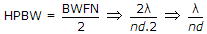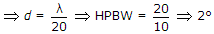.

QUESTION: 3

### Refractive index of glass is 1.5. Find the wavelength of a beam of light with a frequency of 1014 Hz in glass. Assume velocity of light is 3 x 108 m/sec in vacuum.

Solution:

Given, μ = 1.6, f = 1014 Hz, v = 3 x 108 m/sec.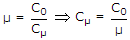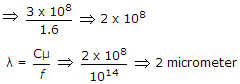QUESTION: 4

The function f(x - vot) represent which of the following?

Solution:

f(x - vot) represents in +ve direction while f(x + vot) represents a progressive wave in -ve direction.

QUESTION: 5

A broadside array operating at 100 cm wavelength consist of 4 half-wave dipoles spaced 50 cm apart. Each element carries radio frequency current in the same phase and of magnitude 0.5 A. The radiated power will be

Solution:

P = n . I2 . Rr, where Rr = 80p2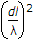Ω.

n = 4.

QUESTION: 6

The input impedance of short-circuited line of length l where λ/4 < l < λ/2, is

Solution:

l < λ/4, ZL = 0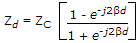, if Zd comes +j kZC then inductive,

If Zd comes -j kZC then capacitive.

for 0 ≤ l ≤ 1/4, λ ≤ l ≤ 3λ/4 will be inductive.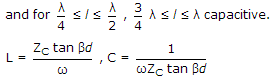QUESTION: 7

A wave is propagated in a waveguide at frequency of 9 GHz and separation is 2 cm between walls find cut off wavelength for dominant mode.

Solution: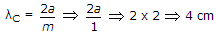.

QUESTION: 8

Find the radiation resistance of an antenna of length λ/10 meter?

Solution: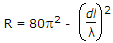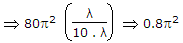.

QUESTION: 9

Charge needed within a unit sphere centred at the origin for producing a potential field,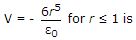Solution: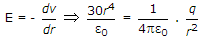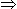q = 120p coulomb, r = 1 meter.

QUESTION: 10

A rectangular metal waveguide filled with a dielectric of relative permittivity εr = 4, has the inside dimensions 3 x 1.2 cm, the cut off frequency for the dominant mode is

Solution: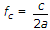for dominant mode.

QUESTION: 11

A wave is propagated in a waveguide at frequency of 9 GHz and separation is 2 cm between walls Calculate group velocity for dominant mode.

Solution:

Vg . Vp = C2

where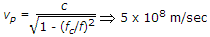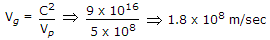.

QUESTION: 12

For F1 layer the maximum ionic density is 2.3 x 104 electrons per cc. The critical frequency for this layer will be

Solution:

fc = 9Nmax .

QUESTION: 13

Which one of the following does represents the electric field lines for the TE02 mode in the cross section of a hollow rectangular metallic waveguide?

Solution:

TE02m = 0, n = 2

in x direction no propagation, only in y, and normal to the waveguide, tangential component is continuous.

QUESTION: 14

The velocity of electromagnetic wave in a good conductor is

Solution: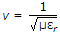, for good conductor εr >> 1.

QUESTION: 15

Radiation intensity of a dipole depends strongly on frequency. If at a frequency f, the intensity of radiation is 'I'. Then at a frequency of f/2, the intensity will be

Solution:

I ∝ f.

QUESTION: 16

Consider a 300 Ω, quarter wave long at 1 GHz transmission line as shown in figure. It is connected to a 10 V, 50 Ω source at one end is left open circuited at the other end. The magnitude of the voltage at the open circuit end of the line is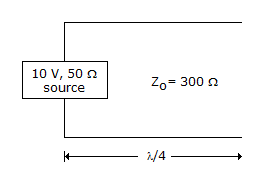Solution:

γ = a + jβ a = 0γ = jβ

Propagating wave

v = VI e-rz + VR erz

No change in the voltage at the open end it will same,

vr = 10 volt.

QUESTION: 17

The phase angle corresponding to λ/4 in a standing-wave pattern is

Solution: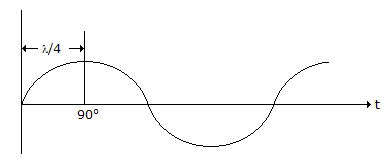QUESTION: 18

A hollow rectangular waveguide has dimensions a = 2b. Calculate the amount of attenuation, if the frequency is 3 GHz, and b = 1 cm.

Solution: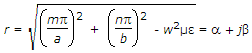Since, wave is attenuated hence β = 0

and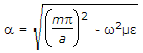Take, dominant mode m = 1,

n = 0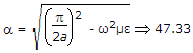.

QUESTION: 19

Calculate the directivity of an antenna for θE = 30º, θH = 60

Solution: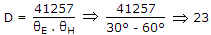.

QUESTION: 20

Most antenna consisting of a 50 meter long vertical conductor operates over a perfectly conducting ground plane. It is base fed at a frequency of 600 kHz. The radiation resistance of the antenna in ohms is

Solution: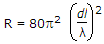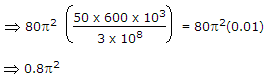QUESTION: 21

A (75 - j 40) W load is connected to a co-axial line of z0 = 75ω at 6 MHz. The load matching on the line can be accomplished by connecting

Solution:

ZL = 75 - J . 40, ZC = 75 W, f = 6 mHz

Given ZL is capacitive, so proper matching, load must be inductive.

QUESTION: 22

A plane wave is characterized by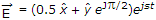the wave is

Solution:

Because, magnitude is different (0.5, 1) and phase is 90º

QUESTION: 23

The electric field on the surface of a perfect conductor is 2V/m. The conductor is immersed in water with ε = 80 ε0. The surface charge density on the conductor is ((ε0 = 10-9/36p)F/m)

Solution: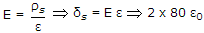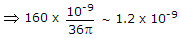.

QUESTION: 24

A wave is propagated in a waveguide at frequency of 9 GHz and separation is 2 cm between walls calculate the phase velocity for dominant mode.

Solution:

Vg . Vp = C2

where.

QUESTION: 25

A plane electromagnetic wave travels in dielectric medium of relative permittivity 9. Relative to free space, the velocity of propagation in the dielectric is

Solution: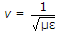.